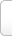﻿ 个组词_个字可以怎么组词_能组什么词_有哪些

# 个 字可以怎么组词

• 个人经济
• 个人利益最大化
• 个人风格
• 个泡
• 个人自由
• 个股
• 个别
• 个人养老保险
• 个中人
• 个贷
• 个人发展
• 个人利益
• 个把
• 个别活动
• 个体工商户
• 个性化
• 个人理想
• 个税
• 个人主义
• 个人隐私
• 个体性
• 个性难改
• 个体户
• 个性
• 个人需求
• 个人电脑
• 个体经营者
• 个人意识
• 个事
• 个中滋味
• 个人能力
• 个体发生
• 个人兴趣
• 个案研究
• 个个
• 个案
• 个案实验
• 个人简介
• 个人制度
• 个人修养
• 个股分化
• 个体
• 个别教学
• 个人财产
• 个人简历
• 个位
• 个人所得税起征点
• 个人所得
• 个性观察
• 个人尊严
• 个别差异
• 个性与共性
• 个调税
• 个人爱好
• 个人行为
• 个子
• 个数
• 个人魅力
• 个人价值
• 个体医
• 个儿
• 个人游
• 个体经济
• 个人企业
• 个人空间
• 个头
• 个人诉求
• 个人卫生
• 个中
• 个人主义者
• 个体社会化
• 个人破产制度

## “个”字在中间的能组哪些词

• 第七个十字架
• 一个萝卜一个坑
• 一个人
• 到哪座山里唱哪个歌
• 共性与个性
• 打个花
• 一个月亮
• 德国--一个冬天的童话
• 七个头八个胆
• 行个方便
• 赔个不是
• 爱一个人
• 三个臭皮匠
• 无个事
• 一个巴掌拍不响
• 生个子
• 有个数儿
• 自个儿
• 有个好歹
• 一个中心，两个基本点
• 一步一个脚印
• 半个前程
• 一个样儿
• 掉个儿
• 隔里个里
• 打个问号
• 两个肩膀扛张嘴
• 四个字
• 一个子儿
• 一个劲儿
• 身个子
• 两个字
• 卖个破绽
• 一个都不能少
• 三个臭皮匠顶个诸葛亮
• 吃个虱子留只脚
• 这个当儿
• 比个高下
• 三个字
• 四个意识
• 一点水一个泡
• 各个击破
• 换个儿
• 有个人
• 打个照面
• 每个人
• 四个全面
• 扑个空
• 不是个儿
• 一般与个别
• 讨个分晓
• 一个朋友
• 一央一个肯
• 一步八个谎
• 挨个儿
• 四个一
• 一问一个肯
• 翻个儿
• 告个幺二三
• 三个臭皮匠，合成一个诸葛亮
• 一个劲
• 打个照会
• 啷个哩个啷
• 成个儿
• 攀个大

## “个”字在结尾组词有哪些

• 夜里个
• 右个
• 一个半个
• 今日个
• 一递一个
• 一个是一个
• 能个
• 若个
• 咱个
• 今儿个
• 十二个
• 灭不个
• 只个
• 通个
• 各个
• 这些个
• 者个
• 之个
• 咋个
• 来得个
• 挨个
• 那些个
• 浑个
• 朗个
• 最后一个
• 前儿个
• 满好个
• 左个
• 子个
• 今个
• 自个
• 麦个
• 无个
• 顶个
• 某个
• 个个
• 真个
• 另一个
• 堂个
• 翻个
• 些个
• 你个
• 哪个
• 几个
• 赶明儿个
• 昨儿个
• 夜儿个
• 好些个
• 好个
• 一个
• 这个
• 整个
• 遮个
• 又弱一个
• 一百个
• 半个
• 明儿个
• 多个
• 那个
• 逐个
• 全个
• 则个
• 七个八个
• 这般个
• 此个
• 三个
• 赶明个

### 个 的拼音及解释Tip:SCCG